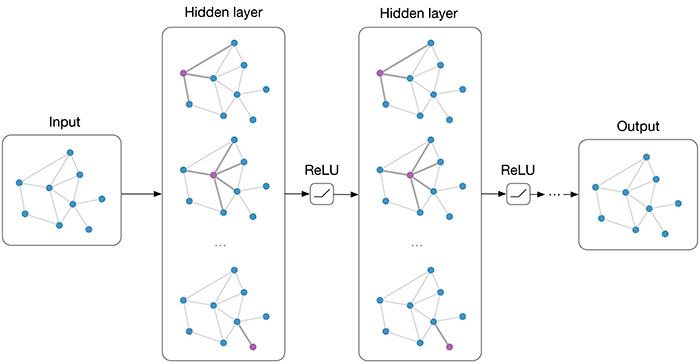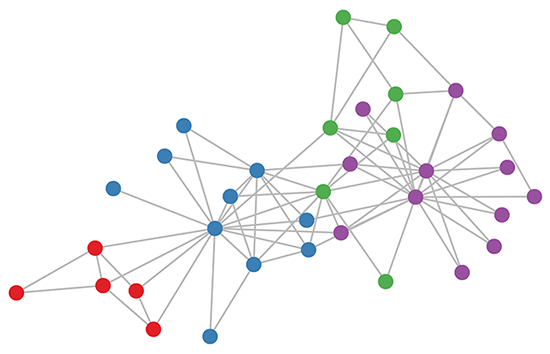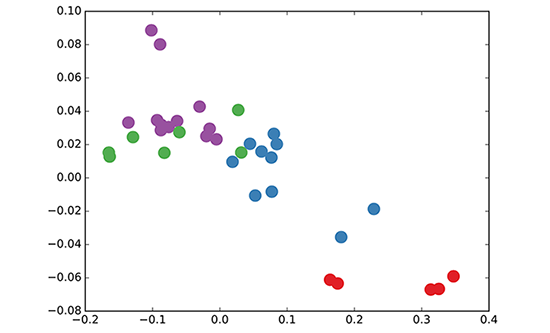## Graph Convolutional Networks

Thomas Kipf, 30 September 2016Multi-layer Graph Convolutional Network (GCN) with first-order filters.

### Overview

Many important real-world datasets come in the form of graphs or networks: social networks, knowledge graphs, protein-interaction networks, the World Wide Web, etc. (just to name a few). Yet, until recently, very little attention has been devoted to the generalization of neural network models to such structured datasets.

In the last couple of years, a number of papers re-visited this problem of generalizing neural networks to work on arbitrarily structured graphs (Bruna et al., ICLR 2014; Henaff et al., 2015; Duvenaud et al., NIPS 2015; Li et al., ICLR 2016; Defferrard et al., NIPS 2016; Kipf & Welling, ICLR 2017), some of them now achieving very promising results in domains that have previously been dominated by, e.g., kernel-based methods, graph-based regularization techniques and others.

In this post, I will give a brief overview of recent developments in this field and point out strengths and drawbacks of various approaches. The discussion here will mainly focus on two recent papers:

and a review/discussion post by Ferenc Huszar: How powerful are Graph Convolutions? that discusses some limitations of these kinds of models. I wrote a short comment on Ferenc's review here (at the very end of this post).

### Outline

• Short introduction to neural network models on graphs
• Spectral graph convolutions and Graph Convolutional Networks (GCNs)
• Demo: Graph embeddings with a simple 1st-order GCN model
• GCNs as differentiable generalization of the Weisfeiler-Lehman algorithm

If you're already familiar with GCNs and related methods, you might want to jump directly to Embedding the karate club network.

### How powerful are Graph Convolutional Networks?

#### Recent literature

Generalizing well-established neural models like RNNs or CNNs to work on arbitrarily structured graphs is a challenging problem. Some recent papers introduce problem-specific specialized architectures (e.g. Duvenaud et al., NIPS 2015; Li et al., ICLR 2016; Jain et al., CVPR 2016), others make use of graph convolutions known from spectral graph theory1 (Bruna et al., ICLR 2014; Henaff et al., 2015) to define parameterized filters that are used in a multi-layer neural network model, akin to "classical" CNNs that we know and love.

More recent work focuses on bridging the gap between fast heuristics and the slow2, but somewhat more principled, spectral approach. Defferrard et al. (NIPS 2016) approximate smooth filters in the spectral domain using Chebyshev polynomials with free parameters that are learned in a neural network-like model. They achieve convincing results on regular domains (like MNIST), closely approaching those of a simple 2D CNN model.

In Kipf & Welling (ICLR 2017), we take a somewhat similar approach and start from the framework of spectral graph convolutions, yet introduce simplifications (we will get to those later in the post) that in many cases allow both for significantly faster training times and higher predictive accuracy, reaching state-of-the-art classification results on a number of benchmark graph datasets.

#### GCNs Part I: Definitions

Currently, most graph neural network models have a somewhat universal architecture in common. I will refer to these models as Graph Convolutional Networks (GCNs); convolutional, because filter parameters are typically shared over all locations in the graph (or a subset thereof as in Duvenaud et al., NIPS 2015).

For these models, the goal is then to learn a function of signals/features on a graph $$\mathcal{G}=(\mathcal{V}, \mathcal{E})$$ which takes as input:

• A feature description $$x_i$$ for every node $$i$$; summarized in a $$N\times D$$ feature matrix $$X$$ ($$N$$: number of nodes, $$D$$: number of input features)
• A representative description of the graph structure in matrix form; typically in the form of an adjacency matrix $$A$$ (or some function thereof)

and produces a node-level output $$Z$$ (an $$N\times F$$ feature matrix, where $$F$$ is the number of output features per node). Graph-level outputs can be modeled by introducing some form of pooling operation (see, e.g. Duvenaud et al., NIPS 2015).

Every neural network layer can then be written as a non-linear function $H^{(l+1)} = f(H^{(l)}, A) \, ,$ with $$H^{(0)} = X$$ and $$H^{(L)} = Z$$ (or $$z$$ for graph-level outputs), $$L$$ being the number of layers. The specific models then differ only in how $$f(\cdot, \cdot)$$ is chosen and parameterized.

#### GCNs Part II: A simple example

As an example, let's consider the following very simple form of a layer-wise propagation rule:

$f(H^{(l)}, A) = \sigma\left( AH^{(l)}W^{(l)}\right) \, ,$

where $$W^{(l)}$$ is a weight matrix for the $$l$$-th neural network layer and $$\sigma(\cdot)$$ is a non-linear activation function like the $$\text{ReLU}$$. Despite its simplicity this model is already quite powerful (we'll come to that in a moment).

But first, let us address two limitations of this simple model: multiplication with $$A$$ means that, for every node, we sum up all the feature vectors of all neighboring nodes but not the node itself (unless there are self-loops in the graph). We can "fix" this by enforcing self-loops in the graph: we simply add the identity matrix to $$A$$.

The second major limitation is that $$A$$ is typically not normalized and therefore the multiplication with $$A$$ will completely change the scale of the feature vectors (we can understand that by looking at the eigenvalues of $$A$$). Normalizing $$A$$ such that all rows sum to one, i.e. $$D^{-1}A$$, where $$D$$ is the diagonal node degree matrix, gets rid of this problem. Multiplying with $$D^{-1}A$$ now corresponds to taking the average of neighboring node features. In practice, dynamics get more interesting when we use a symmetric normalization, i.e. $$D^{-\frac{1}{2}}AD^{-\frac{1}{2}}$$ (as this no longer amounts to mere averaging of neighboring nodes). Combining these two tricks, we essentially arrive at the propagation rule introduced in Kipf & Welling (ICLR 2017):

$f(H^{(l)}, A) = \sigma\left( \hat{D}^{-\frac{1}{2}}\hat{A}\hat{D}^{-\frac{1}{2}}H^{(l)}W^{(l)}\right) \, ,$

with $$\hat{A} = A + I$$, where $$I$$ is the identity matrix and $$\hat{D}$$ is the diagonal node degree matrix of $$\hat{A}$$.

In the next section, we will take a closer look at how this type of model operates on a very simple example graph: Zachary's karate club network (make sure to check out the Wikipedia article!).

#### GCNs Part III: Embedding the karate club networkKarate club graph, colors denote communities obtained via modularity-based clustering (Brandes et al., 2008).

Let's take a look at how our simple GCN model (see previous section or Kipf & Welling, ICLR 2017) works on a well-known graph dataset: Zachary's karate club network (see Figure above).

We take a 3-layer GCN with randomly initialized weights. Now, even before training the weights, we simply insert the adjacency matrix of the graph and $$X = I$$ (i.e. the identity matrix, as we don't have any node features) into the model. The 3-layer GCN now performs three propagation steps during the forward pass and effectively convolves the 3rd-order neighborhood of every node (all nodes up to 3 "hops" away). Remarkably, the model produces an embedding of these nodes that closely resembles the community-structure of the graph (see Figure below). Remember that we have initialized the weights completely at random and have not yet performed any training updates (so far)!GCN embedding (with random weights) for nodes in the karate club network.

This might seem somewhat surprising. A recent paper on a model called DeepWalk (Perozzi et al., KDD 2014) showed that they can learn a very similar embedding in a complicated unsupervised training procedure. How is it possible to get such an embedding more or less "for free" using our simple untrained GCN model?

We can shed some light on this by interpreting the GCN model as a generalized, differentiable version of the well-known Weisfeiler-Lehman algorithm on graphs. The (1-dimensional) Weisfeiler-Lehman algorithm works as follows3:

For all nodes $$v_i\in \mathcal{G}$$:

• Get features4 $$\{h_{v_j}\}$$ of neighboring nodes $$\{v_j\}$$
• Update node feature $$h_{v_i} \leftarrow \text{hash}\left(\sum_j h_{v_j}\right)$$, where $$\text{hash}(\cdot)$$ is (ideally) an injective hash function

Repeat for $$k$$ steps or until convergence.

In practice, the Weisfeiler-Lehman algorithm assigns a unique set of features for most graphs. This means that every node is assigned a feature that uniquely describes its role in the graph. Exceptions are highly regular graphs like grids, chains, etc. For most irregular graphs, this feature assignment can be used as a check for graph isomorphism (i.e. whether two graphs are identical, up to a permutation of the nodes).

Going back to our Graph Convolutional layer-wise propagation rule (now in vector form):

$h^{(l+1)}_{v_i} = \sigma \left( \sum_{j} \frac{1}{c_{ij}}h^{(l)}_{v_j}W^{(l)} \right) \, ,$

where $$j$$ indexes the neighboring nodes of $$v_i$$. $$c_{ij}$$ is a normalization constant for the edge $$(v_i,v_j)$$ which originates from using the symmetrically normalized adjacency matrix $$D^{-\frac{1}{2}}AD^{-\frac{1}{2}}$$ in our GCN model. We now see that this propagation rule can be interpreted as a differentiable and parameterized (with $$W^{(l)}$$) variant of the hash function used in the original Weisfeiler-Lehman algorithm. If we now choose an appropriate non-linearity and initialize the random weight matrix such that it is orthogonal (or e.g. using the initialization from Glorot & Bengio, AISTATS 2010), this update rule becomes stable in practice (also thanks to the normalization with $$c_{ij}$$). And we make the remarkable observation that we get meaningful smooth embeddings where we can interpret distance as (dis-)similarity of local graph structures!

#### GCNs Part IV: Semi-supervised learning

Since everything in our model is differentiable and parameterized, we can add some labels, train the model and observe how the embeddings react. We can use the semi-supervised learning algorithm for GCNs introduced in Kipf & Welling (ICLR 2017). We simply label one node per class/community (highlighted nodes in the video below) and start training for a couple of iterations5:

Semi-supervised classification with GCNs: Latent space dynamics for 300 training iterations with a single label per class. Labeled nodes are highlighted.

Note that the model directly produces a 2-dimensional latent space which we can immediately visualize. We observe that the 3-layer GCN model manages to linearly separate the communities, given only one labeled example per class. This is a somewhat remarkable result, given that the model received no feature description of the nodes. At the same time, initial node features could be provided, which is exactly what we do in the experiments described in our paper (Kipf & Welling, ICLR 2017) to achieve state-of-the-art classification results on a number of graph datasets.

### Conclusion

Research on this topic is just getting started. The past several months have seen exciting developments, but we have probably only scratched the surface of these types of models so far. It remains to be seen how neural networks on graphs can be further taylored to specific types of problems, like, e.g., learning on directed or relational graphs, and how one can use learned graph embeddings for further tasks down the line, etc. This list is by no means exhaustive and I expect further interesting applications and extensions to pop up in the near future. Let me know in the comments below if you have some exciting ideas or questions to share!

##### Thanks to the following people:

Max Welling, Taco Cohen, Chris Louizos and Karen Ullrich (for many discussions and feedback both on the paper and this blog post). Also I'd like to thank Ferenc Huszar for highlighting some drawbacks of these kinds of models.

##### A note on completeness

This blog post constitutes by no means an exhaustive review of the field of neural networks on graphs. I have left out a number of both recent and older papers to make this post more readable and to give it a coherent story line. The papers that I mentioned here will nonetheless serve as a good start if you want to dive deeper into this topic and get a complete overview of what is around and what has been tried so far.

##### Citation

If you want to use some of this in your own work, you can cite our paper on Graph Convolutional Networks:

@article{kipf2016semi,
title={Semi-Supervised Classification with Graph Convolutional Networks},
author={Kipf, Thomas N and Welling, Max},
journal={arXiv preprint arXiv:1609.02907},
year={2016}
}
##### Source code

We have released the code for Graph Convolutional Networks on GitHub: https://github.com/tkipf/gcn.

###### The issue with regular graphs

In the following, I will briefly comment on the statements made in How powerful are Graph Convolutions?, a recent blog post by Ferenc Huszar that provides a slightly negative view on some of the models discussed here. Ferenc considers the special case of regular graphs. He correctly points out that Graph Convolutional Networks (as introduced in this blog post) reduce to rather trivial operations on regular graphs when compared to models that are specifically designed for this domain (like "classical" 2D CNNs for images). It is indeed important to note that current graph neural network models that apply to arbitrarily structured graphs typically share some form of shortcoming when applied to regular graphs (like grids, chains, fully-connected graphs etc.). A localized spectral treatment (like in Defferrard et al., NIPS 2016), for example, reduces to rotationally symmetric filters and can never imitate the operation of a "classical" 2D CNN on a grid (exluding border-effects). In the same way, the Weisfeiler-Lehman algorithm will not converge on regular graphs. What this tells us, is that we should probably look beyond regular grids when trying to evaluate the usefulness of a specific graph neural network model, as there are specific trade-offs that have to be made when designing such models for arbitary graphs (yet it is of course important to make people aware of these trade-offs) - that is, unless we can come up with a universally powerful model at some point, of course.

1. A spectral graph convolution is defined as the multiplication of a signal with a filter in the Fourier space of a graph. A graph Fourier transform is defined as the multiplication of a graph signal $$X$$ (i.e. feature vectors for every node) with the eigenvector matrix $$U$$ of the graph Laplacian $$L$$. The (normalized) graph Laplacian can be easily computed from the symmetrically normalized graph adjacency matrix $$\tilde{A}$$: $$L = I - \tilde{A}$$.

2. Using a spectral approach comes at a price: Filters have to be defined in Fourier space and a graph Fourier transform is expensive to compute (it requires multiplication of node features with the eigenvector matrix of the graph Laplacian, which is a $$O(N^2)$$ operation for a graph with $$N$$ nodes; computing the eigenvector matrix in the first place is even more expensive).

3. I'm simplifying things here. For an extensive mathematical discussion of the Weisfeiler-Lehman algorithm, have a look at the paper by Douglas (2011).

4. Node features for the Weisfeiler-Lehman algorithm are typically chosen as scalar integers and are often referred to as colors.

5. As we don't anneal the learning rate in this example, things become quite "jiggly" once the model has converged to a good solution.